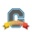cancel
Showing results for
Did you mean:
Surveyor II

## How does drop lowest grade work?

Does it just go by a percentage?  Right now, I have some assignments that are worth slightly different total points.  And dropping the lowest assignment by percentage will not necessarily be the assignment that a student would choose to optimize their score.  As an extreme example, suppose a student currently has a 90% out of 1000pts.  They submit two assignments.  On one, they get 1/2 pts, and on the other they get 700/1000 pts.  Ostensibly, on the 1/2 assignment, they got 50%, so a naive assignment dropping algorithm would drop that grade (since they got a 70% on the other one).  However, if they drop the 1/2, they end up with an 80% in the class, whereas if they drop the 700/1000, they end up with an 89.92%.

If "by percent score" is how the algorithm works, is there any way to override this behavior manually?  How can I specify the correct assignment to drop, as opposed to letting Canvas choose?

1 Solution

Accepted SolutionsCommunity Coach

Greetings,

I normally suggest that people only use the Drop (ignore) lowest score feature if all assignments in the Assignment Group have the same point value.  But, that certainly isn't mandatory and it sounds like you understand the implications of not having them all be the same point value.  Take a look at this page about Rules in assignment groups; the upper sections in the shaded box in particular.  I've pasted below part of it.

Canvas considers how the rule most negatively or positively affects the student's overall score. A rule to drop the lowest score will remove the assignment score(s) from a student's group percentage calculation that will result in the best possible score for that group. A rule to drop the highest score will remove the assignment score(s) from a student's group percentage calculation that will result in the lowest possible score for that group. Dropping both the lowest and the highest score(s) removes any outlying scores and calculates a student’s grade based upon the remaining middle scores.

Dropping scores is based on the impact to the total points for that assignment group. In some cases, the point value may be considered more important than percentage score when determining which assignment to drop. For example, if a student earns 100% on a 50-point assignment, 65% on a 100-point assignment, and 50% on a 24-point assignment, the student’s total score is 127 out of 174 points, or 73% for the assignment group. If an instructor sets a rule to drop the assignment with the lowest score in the assignment group, Canvas will drop the score that gives the student a better total score for the group. Even though the 50% score is the lowest percentage, the assignment with the 65% score will be dropped, giving the student a score of 62 out of 74 points, or an 84%, for the assignment group.

Based on that information I think it should work how you are wanting it to.

RickCommunity Coach

Greetings,

I normally suggest that people only use the Drop (ignore) lowest score feature if all assignments in the Assignment Group have the same point value.  But, that certainly isn't mandatory and it sounds like you understand the implications of not having them all be the same point value.  Take a look at this page about Rules in assignment groups; the upper sections in the shaded box in particular.  I've pasted below part of it.

Canvas considers how the rule most negatively or positively affects the student's overall score. A rule to drop the lowest score will remove the assignment score(s) from a student's group percentage calculation that will result in the best possible score for that group. A rule to drop the highest score will remove the assignment score(s) from a student's group percentage calculation that will result in the lowest possible score for that group. Dropping both the lowest and the highest score(s) removes any outlying scores and calculates a student’s grade based upon the remaining middle scores.

Dropping scores is based on the impact to the total points for that assignment group. In some cases, the point value may be considered more important than percentage score when determining which assignment to drop. For example, if a student earns 100% on a 50-point assignment, 65% on a 100-point assignment, and 50% on a 24-point assignment, the student’s total score is 127 out of 174 points, or 73% for the assignment group. If an instructor sets a rule to drop the assignment with the lowest score in the assignment group, Canvas will drop the score that gives the student a better total score for the group. Even though the 50% score is the lowest percentage, the assignment with the 65% score will be dropped, giving the student a score of 62 out of 74 points, or an 84%, for the assignment group.

Based on that information I think it should work how you are wanting it to.

Rick